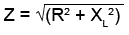# The Impedance Triangle

• After studying this section, you should be able to describe:
• •The Impedance Triangle.
• •Graphical representation of Impedance.
• •Impedance calculations in inductive and capacitive circuits.

The effect of a practical (real) inductor in limiting the flow of current is not solely due to the back emf effect that gives rise to the inductive reactance XL, but is also partly due to the internal resistance of the inductor. As these two causes, occurring in the same component, cannot be physically separated, it is often essential to consider the combined effects of both reactance and resistance on the current through the inductor. The name used to describe this combined opposition to current flow is IMPEDANCE, which is measured in OHMS but has the symbol Z.### Fig 7.1.1 Constructing an Impedance Trianglefrom a LR Phasor Diagram.

This combination of reactance plus resistance is not simply the sum of these quantities. To obtain the impedance of a simple series LR circuit for example, it is first necessary to calculate the PHASOR SUM of the resistance and the reactance. The reason for this can be understood by studying Fig. 7.1.1, which shows a phasor diagram for the circuit re−drawn as a right−angle triangle. The three sides of the triangle represent the three voltage phasors in the circuit VR VL and VS. Because the circuit components are in series, the same current is common to them all.

Considering the voltages VR and VL in Fig 7.1.1, Ohms law states that VR = IR (current multiplied by resistance), therefore VL will equal IXL (current multiplied by reactance)

Now, as Z is the impedance of the total circuit, the total voltage across the circuit, VS = IZ

Because these phasors now form a right angle triangle, Pythagoras´ theorem can be used to relate all these voltage phasors:

VS2 = VR2 + VL2

therefore (Because V = IR etc.)

(IZ)2 = (IR)2 + (IXL)2 or I2Z2 = I2R2 + I2XL2

Next, dividing throughout by I2 eliminates I2 to give Z2 = R2 + XL2

and taking the square root of both sides of this equation gives a formula to calculate Z (the total impedance of the LR circuit).Note: When using this formula on a calculator it is important to remember to use the brackets, or alternatively, to find the sum of R2 + XL2 before using the square root key.### Fig 7.1.2 Impedance Triangle for CR circuits.

Remember that impedances, resistances and reactances are not phasor quantities in themselves. They do affect the voltages and currents, causing these quantities to change their relationships with each other, but as Z, R and X themselves are not varying with time, they are not phasors.

Fig 7.1.2 illustrates the similar relationship that exists in a resistive/capacitive circuit. The voltage phasor diagram for CR circuits can be converted to a right angle triangle and treated in the same way as the LR diagrams in Fig 7.1.1 to give:## Fig 7.1.3 Impedance Triangle for LCR circuits.

Fig 7.1.3 shows a further variation of the Impedance Triangle that can be used to calculate Impedance when resistance (R), Inductance (L) and Capacitance (C) are all present in the circuit, and the total reactance (X) is the difference between the Inductive Reactance (XL) and Capacitive Reactance (XC). In this case the formula for Z becomes:Impedance is just one of the properties that are vital to understanding AC circuits. If you have studied this module and modules 5 (Phase and Phasors) and 6 (Reactance) you should begin to see how these properties work together. To understand how impedance depends on other properties of a circuit, such as reactance and resistance, and see how impedance affects such things as current and voltage, try some calculations using the formulae from these modules in the Module 7 Impedance Quiz